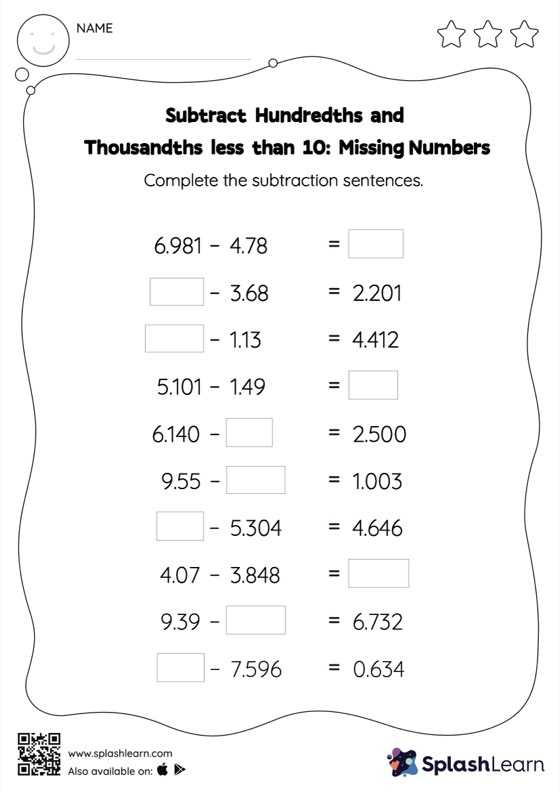# Subtract Hundredths and Thousandths less than 10: Missing Numbers Worksheet

Home > Subtract Hundredths and Thousandths less than 10: Missing NumbersCan your young mathematician subtract hundredths and thousandths less than 10? That's exactly what they do here. Students align the decimal points and use zero as space holders to subtract decimals. Then they use the relationship between addition and subtraction to find the missing number in subtract hundredths and thousandths less than 10 worksheet.